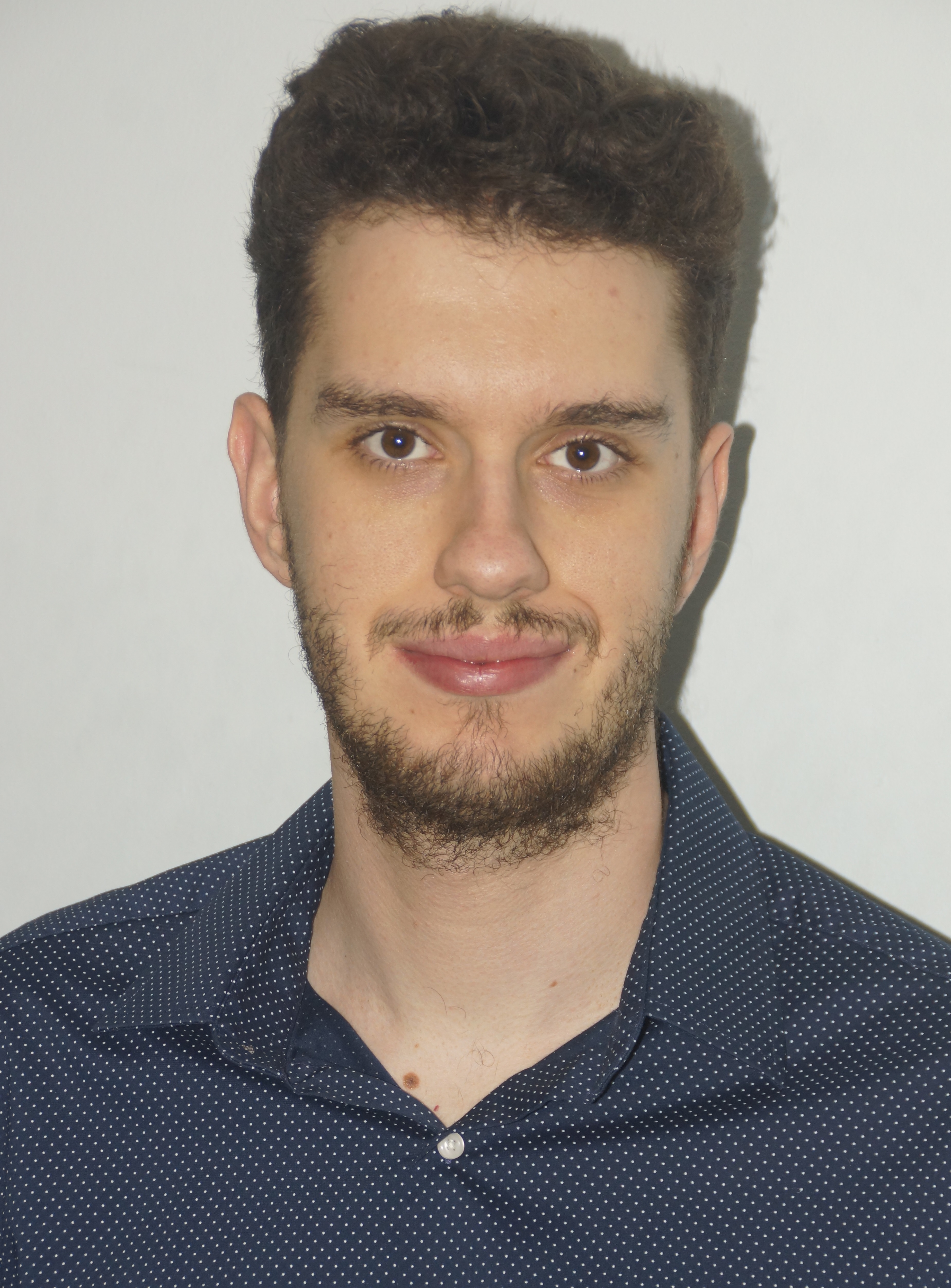Timezone: »

Accelerated Algorithms for Monotone Inclusion and Constrained Nonconvex-Nonconcave Min-Max Optimization
Yang Cai · Argyris Oikonomou · Weiqiang Zheng

We study monotone inclusions and monotone variational inequalities, as well as their generalizations to non-monotone settings. We first show that the \emph{Extra Anchored Gradient (EAG)} algorithm, originally proposed by [Yoon and Ryu, 2021] for unconstrained convex-concave min-max optimization, can be applied to solve the more general problem of Lipschitz monotone inclusion. More specifically, we prove that the EAG solves Lipschitz monotone inclusion problems with an \emph{accelerated convergence rate} of $O(\frac{1}{T})$, which is \emph{optimal among all first-order methods} [Diakonikolas, 2020, Yoon and Ryu, 2021]. Our second result is an {accelerated forward-backward splitting algorithm (AS),} which not only achieves the accelerated $O(\frac{1}{T})$ convergence rate for all monotone inclusion problems, but also exhibits the same accelerated rate for a family of general (non-monotone) inclusion problems that concern negative comonotone operators. As a special case of our second result, AS enjoys the $O(\frac{1}{T})$ convergence rate for solving a non-trivial class of nonconvex-nonconcave min-max optimization problems. Our analyses are based on simple potential function arguments, which might be useful for analysing other accelerated algorithms.

#### Author Information

##### Argyris Oikonomou (Yale University)Argyris Oikonomou is a PhD Student in the department of Computer Science at Yale, advised by Yang Cai. Argyris completed his undergraduate studies at the National Technical University of Athens. His research interests are in mechanism design and algorithmic game theory.